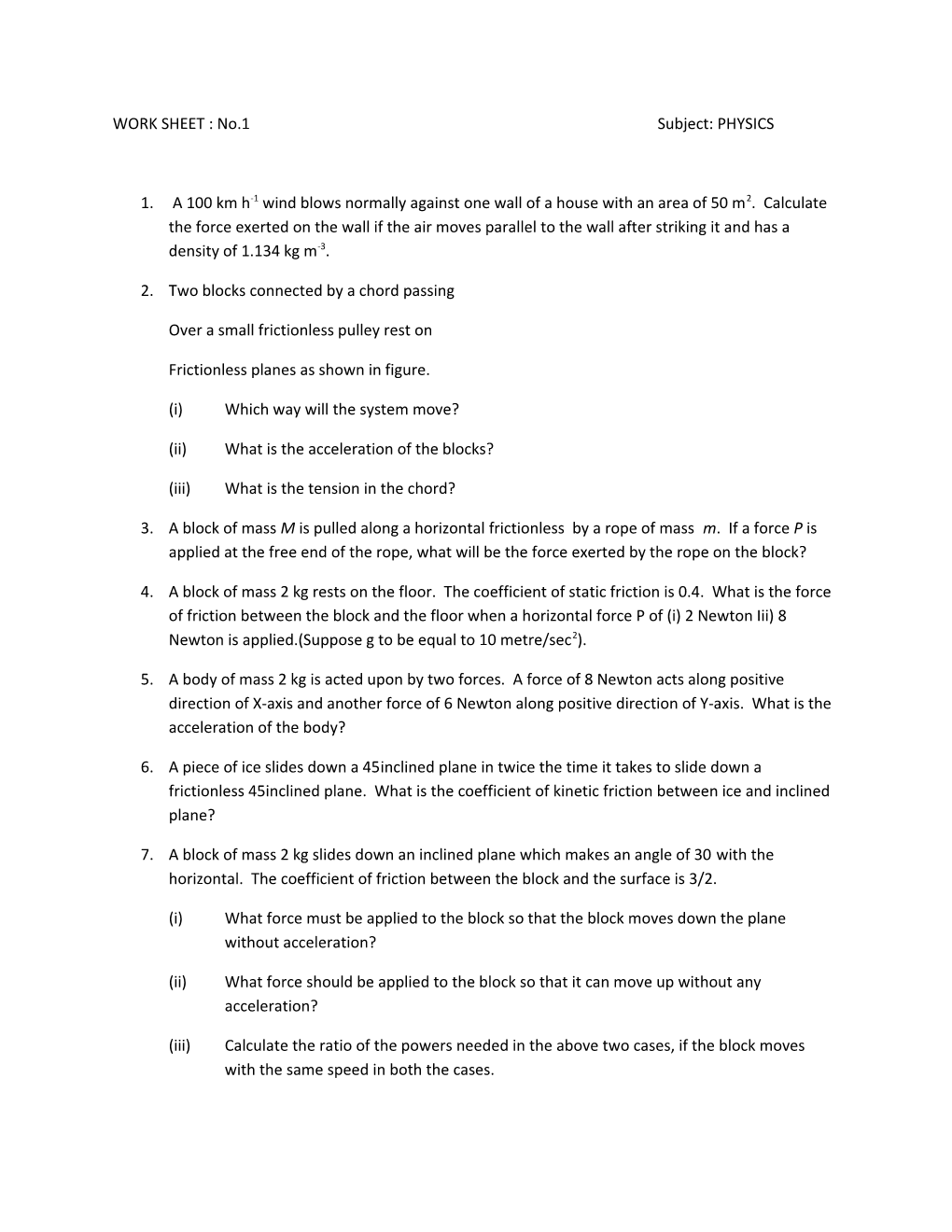# WORK SHEET : No.1 Subject: PHYSICSWORK SHEET : No.1 Subject: PHYSICS

1. A 100 km h-1 wind blows normally against one wall of a house with an area of 50 m2. Calculate the force exerted on the wall if the air moves parallel to the wall after striking it and has a density of 1.134 kg m-3.
2. Two blocks connected by a chord passing

Over a small frictionless pulley rest on

Frictionless planes as shown in figure.

(i)Which way will the system move?

(ii)What is the acceleration of the blocks?

(iii)What is the tension in the chord?

1. A block of mass Mis pulled along a horizontal frictionless by a rope of mass m. If a force Pis applied at the free end of the rope, what will be the force exerted by the rope on the block?
2. A block of mass 2 kg rests on the floor. The coefficient of static friction is 0.4. What is the force of friction between the block and the floor when a horizontal force P of (i) 2 Newton Iii) 8 Newton is applied.(Suppose g to be equal to 10 metre/sec2).
3. A body of mass 2 kg is acted upon by two forces. A force of 8 Newton acts along positive direction of X-axis and another force of 6 Newton along positive direction of Y-axis. What is the acceleration of the body?
4. A piece of ice slides down a 45inclined plane in twice the time it takes to slide down a frictionless 45inclined plane. What is the coefficient of kinetic friction between ice and inclined plane?
5. A block of mass 2 kg slides down an inclined plane which makes an angle of 30 with the horizontal. The coefficient of friction between the block and the surface is 3/2.

(i)What force must be applied to the block so that the block moves down the plane without acceleration?

(ii)What force should be applied to the block so that it can move up without any acceleration?

(iii)Calculate the ratio of the powers needed in the above two cases, if the block moves with the same speed in both the cases.

1. A block of mass 2 kg rests on a plane inclined at an angle of 30 with the horizontal. The coefficient of friction between the block and the surface is 0.7. What will be the force of friction acting on the block?
2. A body of mass 2 kg wt is hung on a spring balance mounted vertically in lift. What will be the reading on the spring balance if the lift descends with an acceleration equal to the acceleration due to gravity `g’?
3. A homogeneous chain of length l lies on a table. What is the maximum length l, of the chain that can hang over the table if the coefficient of friction is µ?
4. A cart with a mass M=1/2 kg is connected

by a string to a weight of mass m= 200 g.

At the initial moment the cart moves to the

Left along a horizontal plane at a speed

Vo=7 ms-1. Find the magnitude and

Direction of the cart, the place where it

Will be and the distance covered by

It in t=5s.

1. Three equal weights A,B,C of mass

2 kg each are hanging on a string

Passing over a fixed pulley which is

Frictionless as shown in the figure.

What will be the tension in the string

Connecting weight B and C?

1. Three blocks A,B and C

Weighing 1,8 and 27 kg respectively

Are connected as shown in the figure

With an inextensible string and are

Moving on a smooth surface. T3

Is equal to 36 Newton. Find T2?

1. Two blocks A and B of masses m1=4 kg

And m2=8 Kg are connected as shown

In figure. Find the force P required to

Move B to the right with uniform

Speed. Disregard the mass of the

Pulley and assume it to be frictionless.

The coefficient of friction between

All surfaces is µ= 0.25.

1. A cart of mass M has a block of mass m attached to it is shown in the figure. The coefficient of friction between the block and the cart is µ. Find the minimum acceleration of the cart so that the block m does not fall?

THE PICTURES FOR SOME QUESTIONS ARE GIEN BELOW:::::